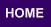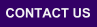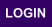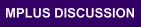Mplus
Sunday
September 24, 2023Mplus Mplus at a GlanceGeneral DescriptionMplus Programs Pricing Version History System Requirements Platforms
 Analyses/ResearchMplus Examples Papers References
 Search

Chi-Square Difference Testing Using the Satorra-Bentler Scaled Chi-Square

Chi-square testing for continuous non-normal outcomes has been discussed in a series of papers by Satorra and Bentler. A popular test statistic is the Satorra-Bentler scaled (mean-adjusted) chi-square, where the usual normal-theory chi-square statistic is divided by a scaling correction to better approximate chi-square under non-normality.

A little-known fact, however, is that such a scaled chi-square cannot be used for chi-square difference testing of nested models because a difference between two scaled chi-squares for nested models is not distributed as chi-square. Mplus issues a warning about this.

In discussions with Albert Satorra, Bengt suggested that Albert might want to figure out how to get a chi-square difference test for the Satorra-Bentler scaled chi-square and he did, producing the following book chapter which can be downloaded as a working paper (in postscript format).

Satorra, A. (2000). Scaled and adjusted restricted tests in multi-sample analysis of moment structures. In Heijmans, R.D.H., Pollock, D.S.G. & Satorra, A. (eds.), Innovations in multivariate statistical analysis. A Festschrift for Heinz Neudecker (pp.233-247). London: Kluwer Academic Publishers.

The formulas in the paper are, however, complex and subsequently Albert and Peter Bentler wrote a paper showing that simple hand calculations using output from nested runs can give the desired chi-square difference test of nested models using the scaled chi-square. This paper is available here: Satorra, A. & Bentler, P.M. (2010). Ensuring positiveness of the scaled difference chi-square test statistic. Psychometrika 75: 243. doi:10.1007/s11336-009-9135-y

Difference Testing Using Chi-Square

Following are the steps needed to compute a chi-square difference test in Mplus using the MLM (Satorra-Bentler), MLR, and WLSM chi-square. DIFFTEST should be used for MLMV and WLSMV. The nested model is the more restrictive model with more degrees of freedom than the comparison model.

1. Compute the difference test scaling correction cd, where d0 is the degrees of freedom in the nested model, c0 is the scaling correction factor for the nested model, d1 is the degrees of freedom in the comparison model, and c1 is the scaling correction factor for the comparison model. Be sure to use the correction factor given in the output for the H0 model.
```      cd = (d0 * c0 - d1*c1)/(d0 - d1)
```
2. Compute the Satorra-Bentler scaled chi-square difference test TRd as follows:
```      TRd = (T0*c0 - T1*c1)/cd
```
where T0 and T1 are the MLM, MLR, or WLSM chi-square values for the nested and comparison model, respectively. For MLM and MLR the products T0*c0 and T1*c1 are the same as the corresponding ML chi-square values.

Difference Testing Using the Loglikelihood

Following are the steps needed to compute a chi-square difference test based on loglikelihood values and scaling correction factors obtained with the MLR estimator.

1. Estimate the nested and comparison models using MLR. The printout gives loglikelihood values L0 and L1 for the H0 and H1 models, respectively, as well as scaling correction factors c0 and c1 for the H0 and H1 models, respectively. For example,
```	L0 = -2,606, c0 = 1.450 with 39 parameters (p0 = 39)
L1 = -2,583, c1 = 1.546 with 47 parameters (p1 = 47)
```
2. Compute the difference test scaling correction where p0 is the number of parameters in the nested model and p1 is the number of parameters in the comparison model.
```	      cd = (p0 * c0 - p1*c1)/(p0 - p1)
= (39*1.450 - 47*1.546)/(39 - 47) = 2.014
```
3. Compute the chi-square difference test (TRd) as follows:
```    	 TRd = -2*(L0 - L1)/cd
= -2*(-2606 + 2583)/2.014 = 22.840
```

Computing the Strictly Positive Satorra-Bentler Chi-Square Difference Test

The robust chi-square difference test can sometimes produce a negative value. An alternative approach that avoids this is given in

Satorra, A., & Bentler, P.M. (2010). Ensuring positiveness of the scaled difference chi-square test statistic. Psychometrika, 75, 243-248.

Mplus Web Note No. 12 shows how to compute this new alternative test.

For an application article, see Bryant and Satorra (2011).